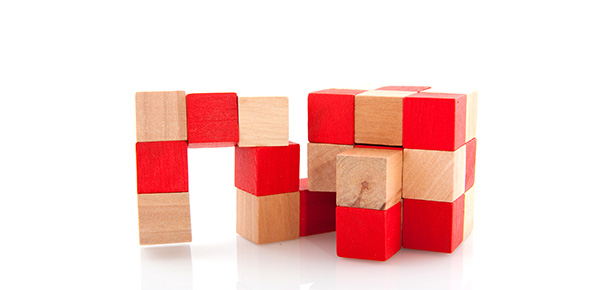# Liquid Volume

10 Questions | Total Attempts: 175SettingsRelated Topics
• 1.
Liquid volume means...
• A.

Measuring a length of a box

• B.

Measuring a person's weight

• C.

Measuring the amount of soda

• D.

Measuring the size of a person

• 2.
1 gallon equals
• A.

2 cups

• B.

4 pints

• C.

4 quarts

• D.

5 cups

• 3.
1 quart equals
• A.

2 gallons

• B.

2 cups

• C.

2 pints

• D.

5 cups

• 4.
1 pint equals
• A.

2 quarts

• B.

2 cups

• C.

2 gallons

• D.

2 pints

• 5.
A can of soda is about..
• A.

A gallon

• B.

A quart

• C.

A pint

• D.

A cup

• 6.
We usually buy milk for our families in ...
• A.

Cups

• B.

Quarts

• C.

Pints

• D.

Gallons

• 7.
What is the biggest size in the US system?
• A.

A cup

• B.

A gallon

• C.

A pint

• D.

A quart

• 8.
What is the smallest size in the US system?
• A.

Gallon

• B.

Quart

• C.

Cup

• D.

Pint

• 9.
A liter is most similar to a...
• A.

Cup

• B.

Quart

• C.

Pint

• D.

Gallon

• 10.
• A.

A baby bottle

• B.

A spoon

• C.

A gas tank in a car

• D.

Soup pot